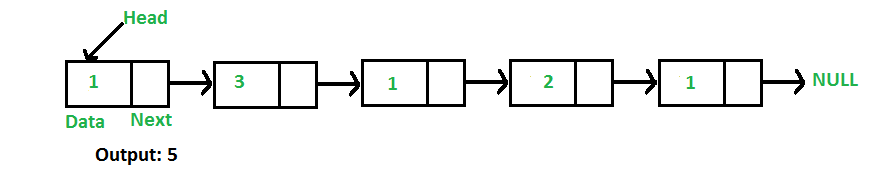# C Program For Finding Length Of A Linked List

• Last Updated : 27 Dec, 2021

Write a function to count the number of nodes in a given singly linked list.For example, the function should return 5 for linked list 1->3->1->2->1.

Iterative Solution:

```1) Initialize count as 0
2) Initialize a node pointer, current = head.
3) Do following while current is not NULL
a) current = current -> next
b) count++;
4) Return count ```

Following is the Iterative implementation of the above algorithm to find the count of nodes in a given singly linked list.

## C

 `// Iterative C program to find length or count ``// of nodes in a linked list``#include``#include`` ` `// Link list node``struct` `Node``{``    ``int` `data;``    ``struct` `Node* next;``};`` ` `/* Given a reference (pointer to pointer) to ``   ``the head of a list and an int, push a new``   ``node on the front of the list. */``void` `push(``struct` `Node** head_ref,  ``          ``int` `new_data)``{``    ``// Allocate node``    ``struct` `Node* new_node =``           ``(``struct` `Node*) ``malloc``(``sizeof``(``struct` `Node));`` ` `    ``// Put in the data ``    ``new_node->data = new_data;`` ` `    ``// Link the old list off the new node``    ``new_node->next = (*head_ref);`` ` `    ``// Move the head to point to the new node``    ``(*head_ref) = new_node;``}`` ` `// Counts no. of nodes in linked list``int` `getCount(``struct` `Node* head)``{``    ``// Initialize count``    ``int` `count = 0;  `` ` `    ``// Initialize current``    ``struct` `Node* current = head;  ``    ``while` `(current != NULL)``    ``{``        ``count++;``        ``current = current->next;``    ``}``    ``return` `count;``}`` ` `// Driver code``int` `main()``{``    ``// Start with the empty list``    ``struct` `Node* head = NULL;`` ` `    ``// Use push() to construct list``    ``// 1->2->1->3->1 ``    ``push(&head, 1);``    ``push(&head, 3);``    ``push(&head, 1);``    ``push(&head, 2);``    ``push(&head, 1);`` ` `    ``// Check the count function``    ``printf``(``"count of nodes is %d"``, ``            ``getCount(head));``    ``return` `0;``}`

Output:

`count of nodes is 5`

Please refer complete article on Find Length of a Linked List (Iterative and Recursive) for more details!

My Personal Notes arrow_drop_up